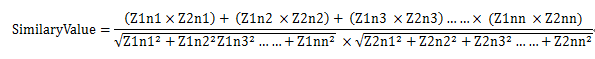### ”分不分开”

• 把一篇文章分割／取出变成若干字符串，然后对结果集合进行相似度匹配，即向量统计法
• 求两篇文章的最大公共子序列(LCS算法)

LCS算法需要根据length(str1)*length(str2)生成矩阵，并进行动态规划计算，空间复杂度和时间复杂度并不理想，得出的最有用结果是两个字符串的最大公共序列（完全相同的最大区域），一小部分对于总体并不是太有价值，所以主流解决方案并未采用，另外一个问题就是不好进行优化，无论如何都要对一篇文章全部分析不能只取出有价值的部分。

LCS实现:

### ”取字还是取特征词”

``````function wordDivide(\$str) {
preg_match_all("/.{1}/u", \$str, \$splitStr);//正则分割，str=>array
return array_count_values(\$splitStr);//统计数组元素频度，array=>dict
}
``````

### ”怎么分析向量集合”

##### LD算法:

Levenshtein Distance，又称编辑距离，指的是两个字符串之间，由一个转换成另一个所需的最少编辑操作次数。根据length(str1)*length(str2)生成矩阵并将之中的元素生成矩阵。

PHP实现:

``````function dummyLevenshtein(\$str0, \$str1)
{
//用矩阵纪录操作数
\$vec = array(); //初始化
for (\$i = 0; \$i <= sizeof(\$str0); \$i++) \$vec[\$i] = \$vec[\$i] = \$i;
for (\$x = 1; \$x <= sizeof(\$str0); \$x++) {
\$src_i = \$str0[\$x - 1];
for (\$y = 1; \$y <= sizeof(\$str1); \$y++) {
\$dst_j = \$str1[\$y - 1];
if (\$src_i == \$dst_j) \$cost = 0; else \$cost = 1; //取左上+当前操作数,左侧+1，上侧+1三个数值中的最小
/
\$vec[\$x][\$y] = min(\$vec[\$x - 1][\$y] + 1, \$vec[\$x][\$y - 1] + 1, \$vec[\$x - 1][\$y - 1] + \$cost);
}
}
return \$vec[sizeof(\$str0)][sizeof(\$str0)];
}

function handleStr(\$str0, \$str1)
{
if (!is_string(\$str0) || !is_string(\$str1)) throw new Exception("Input Error");
if (strlen(\$str0) == 0 || strlen(\$str1) == 0) throw new Exception("Input Error"); //字符串分割
/
preg_match_all("/.{1}/u", \$str0, \$str0);
preg_match_all("/.{1}/u", \$str1, \$str1);
\$str0 = \$str0;
\$str1 = \$str1;
return 1 - (dummyLevenshtein(\$str0, \$str1) * 2 / (sizeof(\$str0) + sizeof(\$str1)));//相似度计算
}
``````

JaccardSimilarity算法:

##### 计算余弦法:PHP实现:

``````//分字
function wordDivide(\$str)
{
if (!is_string(\$str) || strlen(\$str) == 0) {
throw new Exception("Input error!!! ");
}
preg_match_all("/.{1}/u", \$str, \$splitStr);
return array_count_values(\$splitStr);
}

//余弦定理算法
function calcCos(\$wordGroup1, \$wordGroup2)
{
\$unionArray = \$wordGroup1;
//计算并集
foreach (\$wordGroup2 as \$key => \$value)
\$unionArray[\$key] += \$value;
\$denominator = 0;
\$count1 = \$count2 = 0;
//遍历并集
foreach (\$unionArray as \$key => \$value) {
\$denominator += \$wordGroup1[\$key] * \$wordGroup2[\$key];
\$count1 += \$wordGroup1[\$key] * \$wordGroup1[\$key];
\$count2 += \$wordGroup2[\$key] * \$wordGroup2[\$key];
}
//返回余弦结果
return \$denominator / sqrt(\$count1 * \$count2);
}

function testStr(\$str1, \$str2)
{
try {
\$str1 = wordDivide(\$str1);
\$str2 = wordDivide(\$str2);
\$back = calcCos(\$str1, \$str2);
echo "OutPut : ", \$back * 100, "%n";
} catch (Exception \$e) {
echo "Error occurred :", \$e;
}
}
``````

### 总之呢

———OSC居然不让外链，好吧是在下输了———–

3

TF-IDF与余弦相似性的应用 : http://www.ruanyifeng.com/blog/2013/03/cosine_similarity.html